# jerry_fuyi

• 随笔 - 92
• 文章 - 0
• 评论 - 31

## 摇摇棒，理工男的择偶权（上）

### 系列概述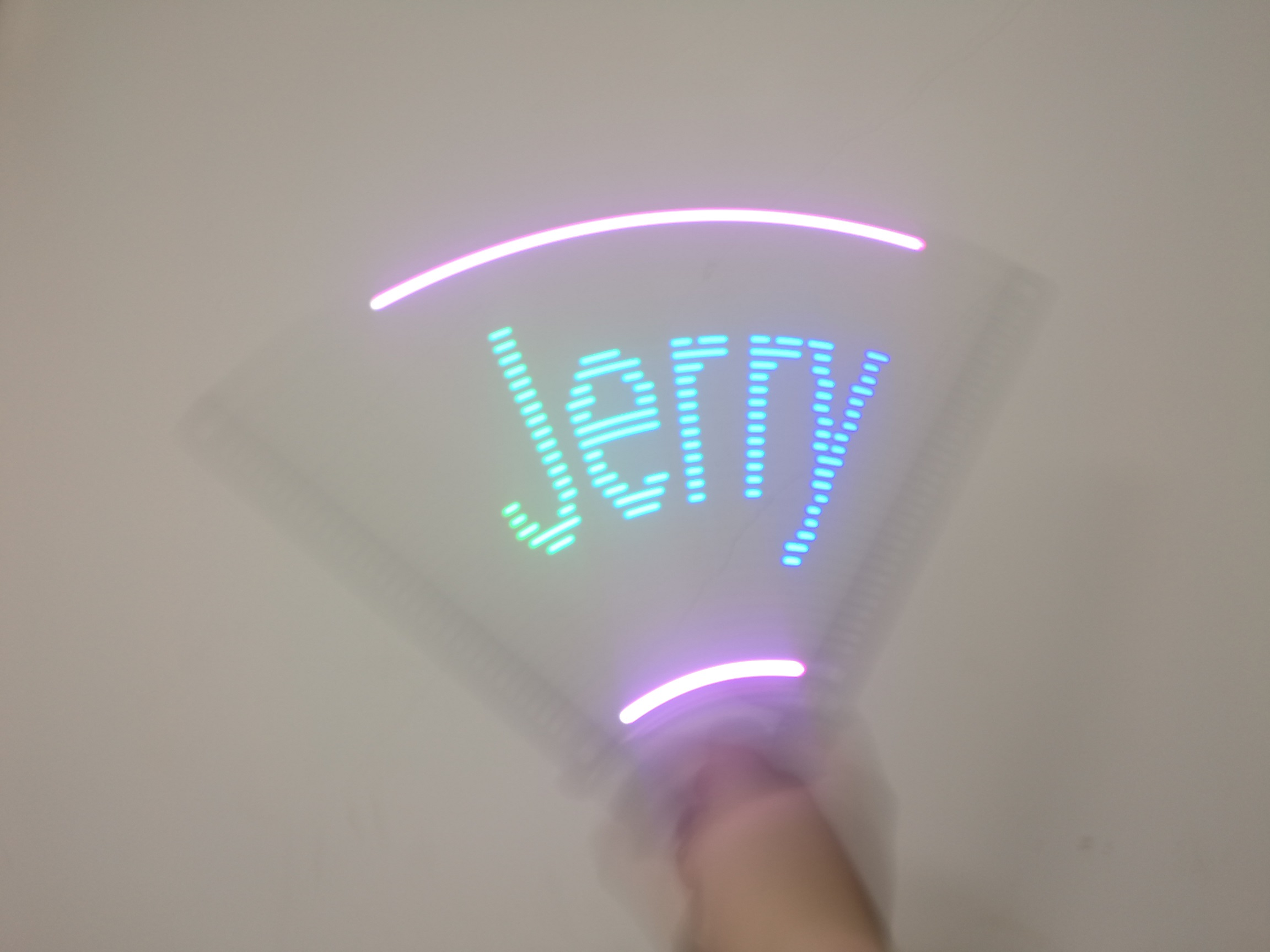### 核心原理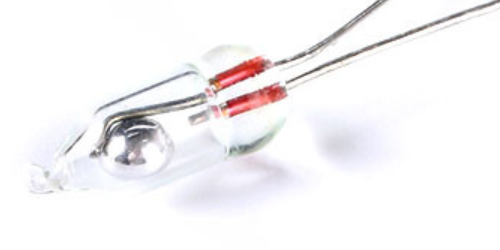### 硬件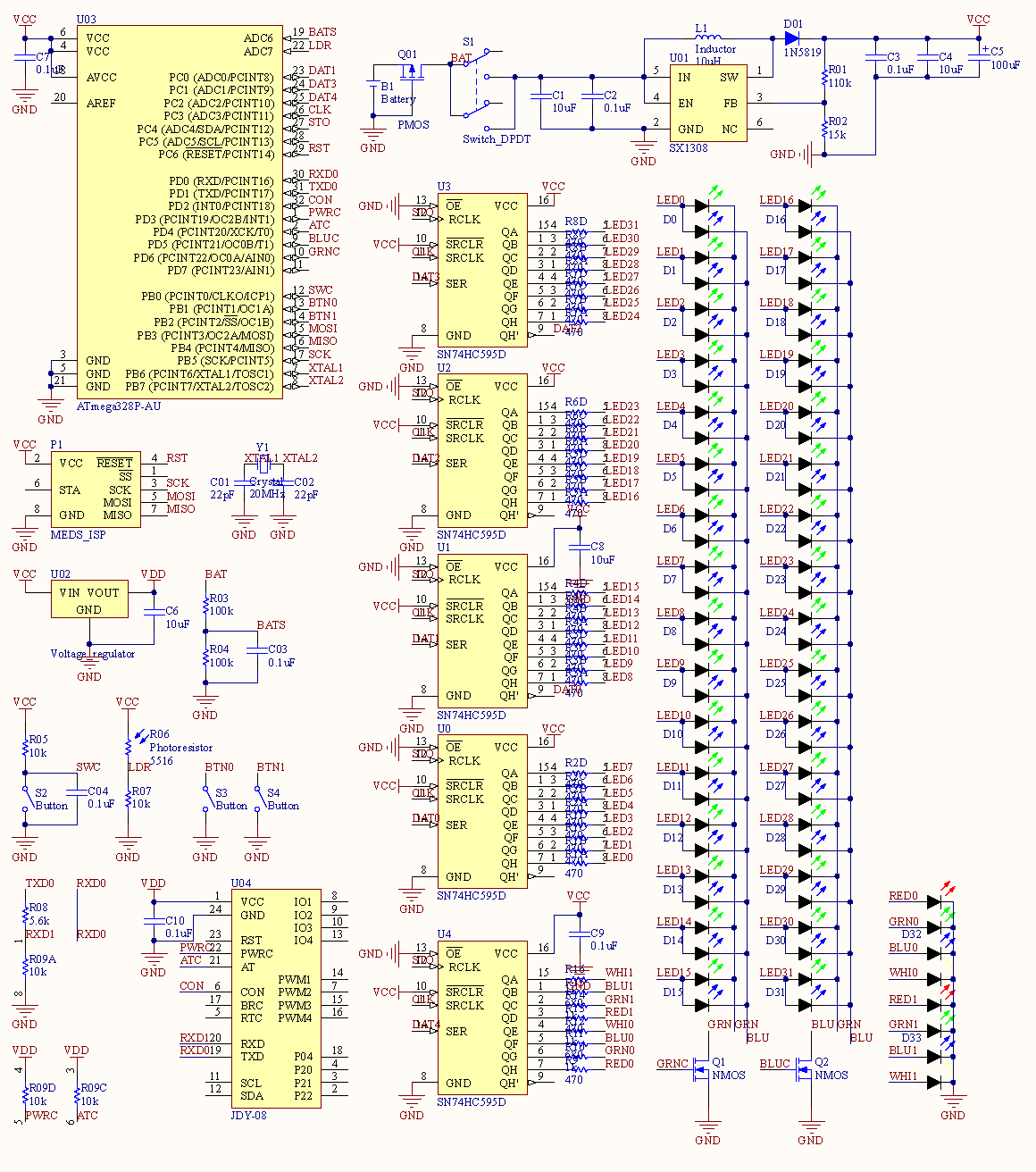• 供电：18650电池座、电源开关、SX1308（B628）升压、AMS1117-3.3稳压；

摇摇棒1.0直接用3.7V锂离子电池供电，但实际电压为2.7V到4.2V，亮度差异很大；2.0的供电部分先升压到5V，为了便于在高亮度下控制亮度。

蓝牙模块需要3.3V电源，所以加了个LDO。

• 控制：ATMega328P单片机、晶振、ISP下载接口；

单片机选择的是我最擅长的AVR系列中的ATmega328P，与烂大街的Arduino相同（但我没从那边抄过哪怕一行代码）。晶振是20MHz的，官方允许的最高频率，为了获得更好的性能。

下载器接口是我自己定义的ISP接口，比标准的占用更少空间，但毕竟是非标准的，这是个历史遗留问题。

• 输入：电池电压检测、水银开关、光敏电阻、按键×2；

水银开关接通时，SWC为低电平；断开时，由于没有负载，SWC为高电平；R05称为上拉电阻。这就是那个很简单的电路。电容C04本来想用于滤波的，实测反而碍事，拿掉了。两个按键同理，上拉电阻在单片机内部配置。

光敏电阻R06阻值与光强负相关，与定值电阻R07分压后的输出电压与光强正相关，接到单片机的ADC（模-数转换器）上，从而检测环境光强度并调整亮度，深夜写代码与阳光下展（liào）示（mèi）都能适配。

• 输出：5片74HC595、2个N沟道MOS管、32个蓝绿双色LED、2个RGBW LED；

595是串行转并行芯片，MOS是一种三极管，详见AVR单片机教程——矩阵键盘。595输出串联排阻后接LED再接到MOS管，连接方式下面细说。

单片机上DAT1DAT3DAT4CLKSTO引脚控制595，前3个是数据信号。设计3个数据信号是为了加速输出，不过最快的输出方式是用SPI，没有用它是设计上的失误。

• 蓝牙：蓝牙模块、简单的电平转换电路。

中篇内容，跳过。

595输出串联电阻后接LED，输出低电平时LED不亮，高电平时有电流因而亮，电阻起到限流作用。不同颜色的灯串联不同阻值的电阻是为了平衡亮度，在RGB都点亮时颜色接近白色。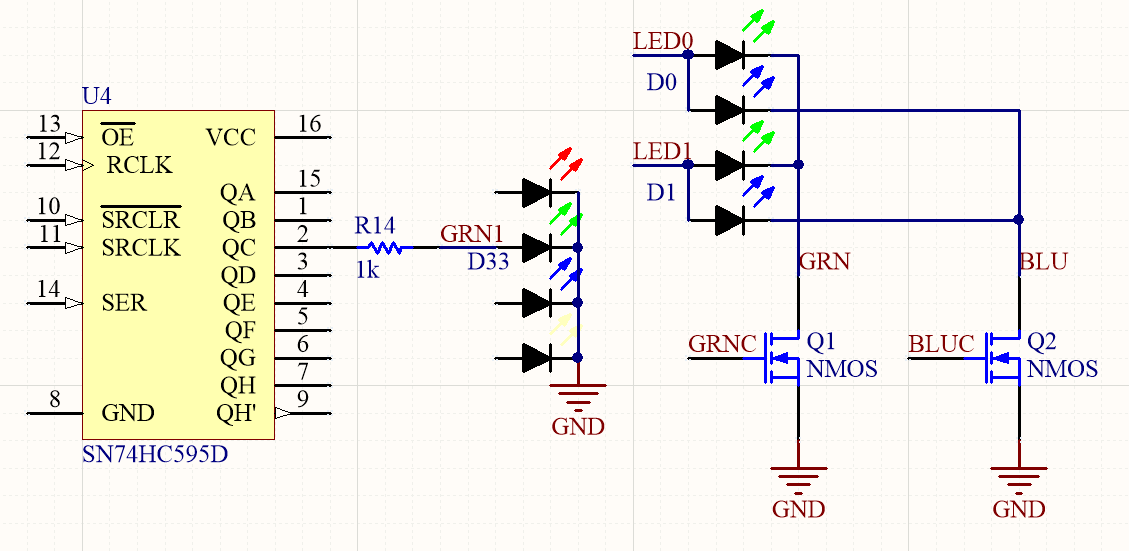4片595输出LED0LED31，越上方的编号越小。每个蓝绿双色LED的两个阳极共同连接一个LEDx信号，绿、蓝阴极分别连接到GRNBLU，是两个MOS管的漏极。当Q的栅极GRNC为高电平时，漏极和连接到GND的源极之间导通，电阻忽略不计，如果此时LEDx为高电平则对应绿灯亮起；低电平时不导通，无论LEDx如何，绿灯一个都不会亮——这段时间留给蓝灯。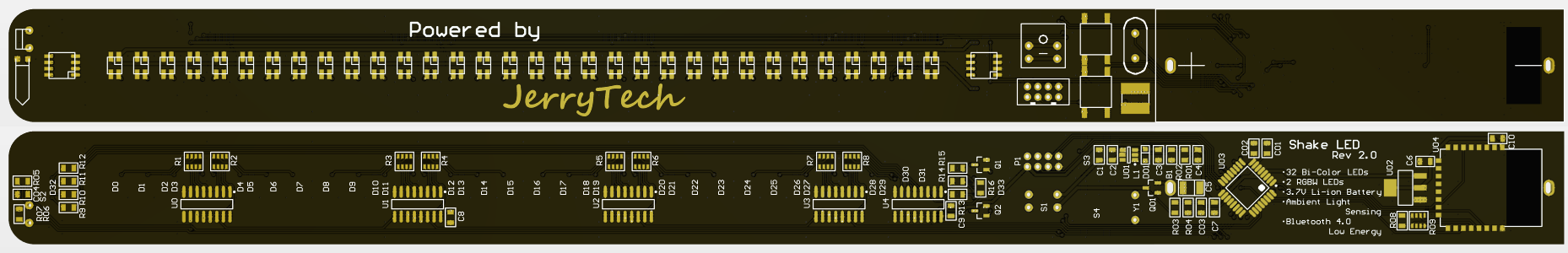PCB渲染图如上。大致布局是，正面最上方水银开关和光敏电阻，往下一个RGBW、32个蓝绿、一个RGBW，IC和电阻等贴片器件都在反面对应的位置。然后是下载器接口、电源开关、两个按键、电感、晶振，最后是电池，反面有升压电路、单片机、蓝牙模块等。在手握摇摇棒时这些元器件会被碰到，影响正常工作，所以全部被我盖了一层热熔胶：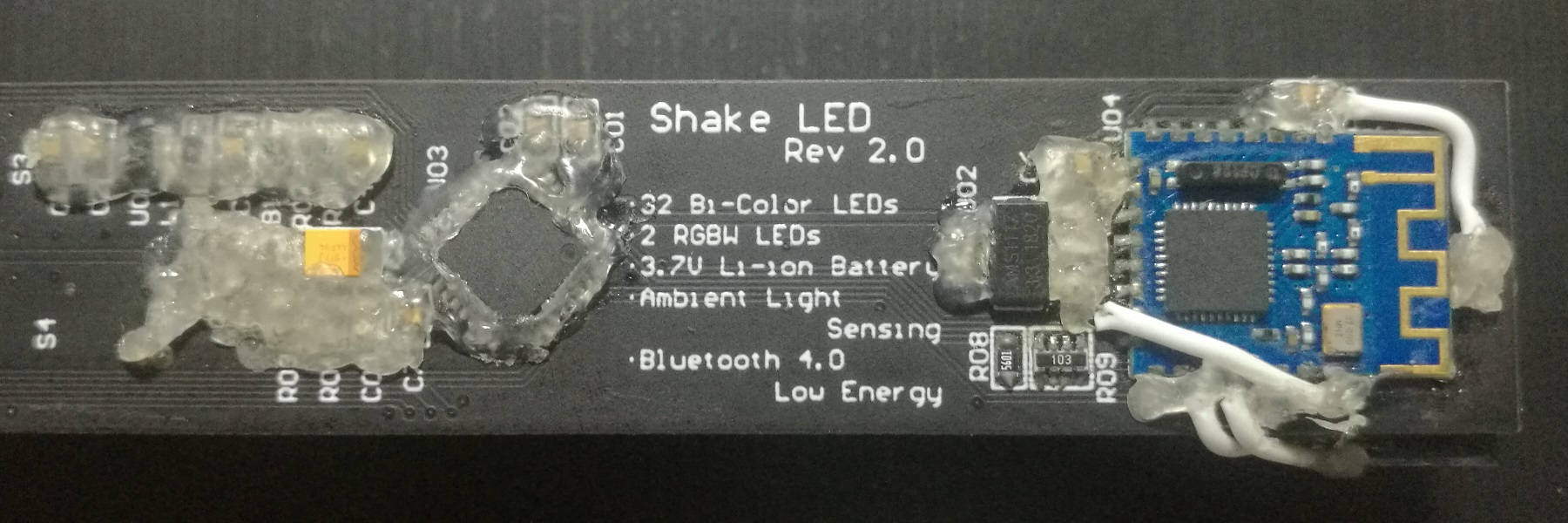• 以任意的蓝绿组合颜色呈现图像，包括渐变色；

• 自动根据环境光强调整显示亮度；

• 用按键切换显示图像、复位周期检测、调整亮度等。

### 驱动

1. LED，规定数据格式，提供以一定亮度亮灯的函数；

2. 水银开关，检测加速度方向，附带滤波；

3. 按键，封装按键双击、长按等高级事件；

5. 定时器，程序结构的核心，定时回调与全局时钟；

6. 蓝牙，依旧跳过。

#### LED

32个双色LED加上2个RGBW的模式可以用5个字节表示，我规定第字节的最低位对应最上方的LED，第字节的最高位对应最下方，第字节最低位对应上方RGBW的红色，最高位对应下方RGBW的白色。这样就不难写出驱动5片595的代码：

uint8_t d0, d1, d2;
d0 = data;
d1 = data;
for (uint8_t i = 0; i != 8; ++i)
{
d0 >>= 1;
d1 >>= 1;
clock_bit(PORTC, 3);
}
d0 = data;
d1 = data;
d2 = data;
for (uint8_t i = 0; i != 8; ++i)
{
d0 >>= 1;
d1 >>= 1;
d2 >>= 1;
clock_bit(PORTC, 3);
}
clock_bit(PORTC, 4);


#define set_bit(r, b) ((r) |= (1u << (b)))
#define reset_bit(r ,b) ((r) &= ~(1u << (b)))
#define read_bit(r, b) ((r) & (1u << (b)))
#define cond_bit(c, r, b) ((c) ? set_bit(r, b) : reset_bit(r, b))
#define flip_bit(r, b) ((r) ^= (1u << (b)))
#define clock_bit(r, b) (flip_bit(r, b), flip_bit(r, b)
#define bit_mask(n, b) (((1u << (n)) - 1) << (b))


typedef enum
{
COLOR_NONE, COLOR_GREEN, COLOR_BLUE
} color_t;

void led_set(color_t color, uint8_t duty, const uint8_t data)
{
// ...
uint8_t com0x;
volatile uint8_t* ocr0x;
switch (color)
{
case COLOR_GREEN:
com0x = 0b10 << COM0A0;
ocr0x = &OCR0A;
break;
case COLOR_BLUE:
com0x = 0b10 << COM0B0;
ocr0x = &OCR0B;
break;
default:
return;
}
if (duty == 0)
return;
TCCR0A |= com0x;
*ocr0x = duty - 1;
TCNT0 = 0xFF;
}


#### 按键

#include <avr/io.h>

#define BUTTON_COUNT 2

static bool pin[BUTTON_COUNT];
static uint8_t filter[BUTTON_COUNT] = {0};

{
switch (which)
{
case 0:
case 1:
}
return false;
}

static inline button_event_t button_filter(uint8_t which)
{
if (which >= BUTTON_COUNT)
return false;
if (now == pin[which])
filter[which] = 0;
else if (++filter[which] == 50)
{
pin[which] = now;
filter[which] = 0;
return now ? BUTTON_LEFT_RELEASED : BUTTON_LEFT_PRESSED;
}
return BUTTON_NONE;
}

void button_init()
{
set_bit(PORTB, 1);
set_bit(PORTB, 2);
for (uint8_t i = 0; i != BUTTON_COUNT; ++i)
}


typedef enum
{
} button_mode_t;

typedef enum
{
BUTTON_NONE,
BUTTON_LEFT_PRESSED, BUTTON_LEFT_RELEASED,
BUTTON_LEFT_SHORT, BUTTON_LEFT_LONG, BUTTON_LEFT_CONT,
BUTTON_LEFT_DOUBLE,
BUTTON_RIGHT_PRESSED, BUTTON_RIGHT_RELEASED,
BUTTON_RIGHT_SHORT, BUTTON_RIGHT_LONG, BUTTON_RIGHT_CONT,
BUTTON_RIGHT_DOUBLE,
BUTTON_BOTH
} button_event_t;


BUTTON_LEFT_CONT指左按键长按以后保持按下的事件，每100毫秒触发一次；BUTTON_BOTH是两个按键同时按下的事件。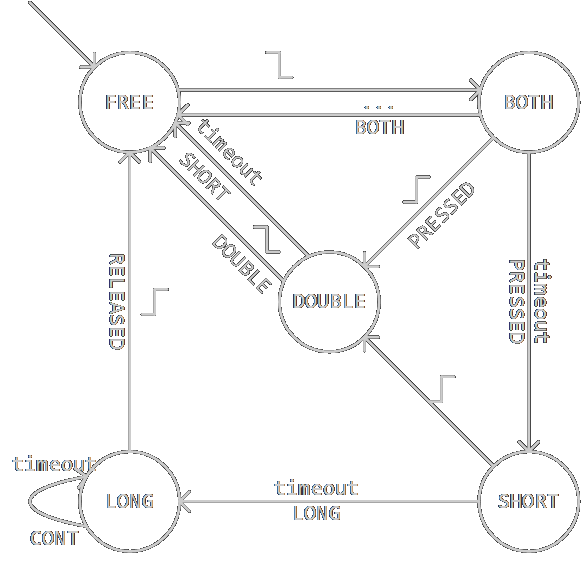BOTHFREE的转移条件为另一个按键也处于BOTH状态。具体timeout值见下面的代码，代码中数值除以5得到毫秒数。

1. 0ms，FREEBOTH

2. 100ms，BOTHSHORT，事件PRESSED

3. 200ms，SHORTDOUBLE

4. 400ms，DOUBLEFREE，事件DOUBLE

typedef enum
{
STATE_FREE, STATE_BOTH, STATE_SHORT, STATE_DOUBLE, STATE_LONG
} state_t;

static button_mode_t mode = MODE_NONE;
static const button_event_t base[BUTTON_COUNT] = {0, BUTTON_RIGHT_PRESSED - BUTTON_LEFT_PRESSED};
static state_t state[BUTTON_COUNT];
static uint16_t count[BUTTON_COUNT];
static uint8_t turn = 0;

void button_mode(button_mode_t m)
{
if (mode == m)
return;
mode = m;
for (uint8_t i = 0; i != BUTTON_COUNT; ++i)
state[i] = STATE_FREE;
}

button_event_t button_get()
{
button_event_t result = BUTTON_NONE;
button_event_t filter = button_filter(turn);
if (mode == MODE_SIMPLE)
result = filter;
{
switch (state[turn])
{
case STATE_FREE:
if (filter == BUTTON_LEFT_PRESSED)
{
state[turn] = STATE_BOTH;
count[turn] = 0;
}
break;
case STATE_BOTH:
{
uint8_t other = 1 - turn;
if (state[other] == STATE_BOTH)
{
result = BUTTON_BOTH;
state[turn] = STATE_FREE;
state[other] = STATE_FREE;
}
else if (filter == BUTTON_LEFT_RELEASED)
{
result = BUTTON_LEFT_PRESSED;
state[turn] = STATE_DOUBLE;
count[turn] = 0;
}
else if (++count[turn] == 500)
{
result = BUTTON_LEFT_PRESSED;
state[turn] = STATE_SHORT;
count[turn] = 0;
}
break;
}
case STATE_SHORT:
if (filter == BUTTON_LEFT_RELEASED)
{
state[turn] = STATE_DOUBLE;
count[turn] = 0;
}
else if (++count[turn] == 2500)
{
result = BUTTON_LEFT_LONG;
state[turn] = STATE_LONG;
count[turn] = 0;
}
break;
case STATE_DOUBLE:
if (filter == BUTTON_LEFT_PRESSED)
{
result = BUTTON_LEFT_DOUBLE;
state[turn] = STATE_FREE;
}
else if (++count[turn] == 500)
{
result = BUTTON_LEFT_SHORT;
state[turn] = STATE_FREE;
}
break;
case STATE_LONG:
if (filter == BUTTON_LEFT_RELEASED)
{
result = BUTTON_LEFT_RELEASED;
state[turn] = STATE_FREE;
}
else if (++count[turn] == 500)
{
result = BUTTON_LEFT_CONT;
count[turn] = 0;
}
break;
}
}
if (result != BUTTON_NONE && result != BUTTON_BOTH)
result += base[turn];
if (++turn == BUTTON_COUNT)
turn = 0;
return result;
}


#### 定时器

#include <avr/io.h>
#include <avr/interrupt.h>

static uint16_t tick = 0;

ISR(TIMER2_COMPA_vect)
{
++tick;
timer_handler();
}

void timer_init()
{
if (0)
TIMER2_COMPA_vect();
TCCR2A = 0b10 << WGM20;
TCCR2B = 0 << WGM22 | 0b010 << CS20;
OCR2A = 249;
TIMSK2 = 1 << OCIE2A;
sei();
}

void clock_reset()
{
tick = 0;
}

uint16_t clock_get()
{
return tick;
}


### 应用程序

typedef struct
{
uint8_t width;
uint8_t height_byte;
const uint8_t* display;
uint8_t in_flash : 1;
uint8_t bright;
uint8_t color;
uint8_t rgbw;
} Config;


display指向点阵数据，共width * height_byte字节，每height_byte字节表示一列，RGBW另存。

#include <stdint.h>
#include <avr/pgmspace.h>

static const uint8_t jiayou[] PROGMEM =
{
0x00, 0x00, 0x00, 0x40, 0x00, 0x40, 0x40, 0x00, 0x20, 0x40, 0x00, 0x18,
0x40, 0x00, 0x07, 0x40, 0xF8, 0x09, 0xFE, 0x1F, 0x08, 0x40, 0x00, 0x10,
0x40, 0x00, 0x30, 0x40, 0x00, 0x18, 0xC0, 0xFF, 0x0F, 0xC0, 0x07, 0x00,
0x00, 0x00, 0x00, 0x00, 0x00, 0x00, 0xC0, 0xFF, 0x7F, 0x40, 0x00, 0x08,
0x40, 0x00, 0x08, 0x40, 0x00, 0x08, 0x40, 0x00, 0x08, 0x40, 0x00, 0x08,
0xC0, 0xFF, 0x3F, 0x40, 0x00, 0x30, 0x00, 0x00, 0x00, 0x00, 0x00, 0x00,
0x00, 0x00, 0x00, 0x80, 0x00, 0x00, 0x00, 0x01, 0x02, 0x00, 0x07, 0x72,
0x08, 0x06, 0x7F, 0x18, 0xE0, 0x01, 0x10, 0x18, 0x00, 0x00, 0x07, 0x00,
0xC0, 0x00, 0x00, 0xC0, 0xFF, 0x7F, 0xC0, 0xFF, 0x7F, 0x40, 0x20, 0x10,
0x40, 0x20, 0x10, 0x40, 0x20, 0x10, 0xFE, 0xFF, 0x1F, 0xFE, 0xFF, 0x1F,
0x40, 0x20, 0x10, 0x40, 0x20, 0x10, 0x40, 0x20, 0x10, 0xC0, 0xFF, 0x7F,
0xC0, 0xFF, 0x3F, 0x00, 0x00, 0x00, 0x00, 0x00, 0x00, 0x00, 0x00, 0x00,
};


（点阵数据可用PCtoLCD2002生成；原谅我用拼音命名变量。）

memcpy_P(display.current, display.ptr + display.phase * display.height_byte, display.height_byte);


int main()
{
startup();
while (1)
;
}


#### 周期检测typedef enum
{
MERCURY_NONE, MERCURY_LEFT, MERCURY_RIGHT
} mercury_event_t;

static bool status;
static uint16_t count = 0;

{
}

void mercury_init()
{
}

mercury_event_t mercury_get()
{
if (now == status)
count = 0;
else if (++count == 100)
{
status = now;
count = 0;
return now ? MERCURY_RIGHT : MERCURY_LEFT;
}
return MERCURY_NONE;
}


1. 周期长度大于等于前一周期的0.75倍并且小于1.5倍，这意味着当前周期和上一周期差不多长，用户在稳定地摇动。把period设为两个周期的平均值，这样可以允许周期缓慢变化。

2. 周期长度小于0.75倍，这可能是噪音导致的，应该忽略，不复位时钟。但是这种情况连续出现很多次就不对了，用count记录次数，达到一定值时要进入不稳定状态。这个值被设置为2。

3. 周期长度大于等于1.5倍，用户停止了摇动，直接进入不稳定状态。事实上停止摇动后LED还会闪一下，因为不免存在抖动，导致程序又判定出一个周期。

bool stable;
uint16_t period;
uint8_t count;
bool half_flag;
uint8_t half_count;

void timer_handler()
{
// ...
uint16_t clock = clock_get();
if (mercury_get() == MERCURY_LEFT)
{
if (stable)
{
if (clock < period * 3 / 4)
{
if (++count == 2)
{
stable = false;
count = 0;
}
if (period * 3 / 8 < clock && clock < period * 5 / 8)
{
half_flag = true;
}
}
else if (clock < period * 3 / 2)
{
clock_reset();
if (count == 1 && half_flag)
{
if (++half_count == 2)
{
half_count = 0;
clock = 0;
}
}
else
{
half_count = 0;
}
period = (period + clock) / 2;
count = 0;
half_flag = false;
clock = 0;
}
else
{
stable = false;
count = 0;
}
}
else
{
clock_reset();
if (period / 2 < clock && clock < period * 3 / 2)
{
if (++count == 2)
{
stable = true;
period = (period + clock) / 2;
count = 0;
half_flag = false;
half_count = 0;
clock = 0;
}
}
else
{
period = clock;
count = 0;
}
}
}
// ...
}


#### 性能优化

mega系列有双周期硬件乘法器，但没有硬件除法器，除数确定的除法编译器会转化为乘法来计算，不确定的就只能调用除法路径了。这种除法偶尔算一次还行，每个定时器周期都算就会严重拖慢速度，比如这句判断是否该切换列的语句：

if (clock == period * 3 / 8 + (uint32_t)period * phase / width / 4)
// ...


struct
{
uint16_t threshold_low;
uint16_t threshold_high;
uint16_t half_low;
uint16_t half_high;
uint16_t clock_base;
uint16_t clock_step;
uint16_t clock_compare;
uint16_t green_step;
uint16_t blue_step;
uint8_t rgbw_duty;
} compute;


clock开头的三个变量就是用来优化前述语句的。在显示周期开始，即clock == 0时，先计算：

compute.clock_base = motion.period * 3 / 8;
compute.clock_step = motion.period / display.width;
compute.clock_compare = compute.clock_base;


compute.clock_compare就是if中与clock比较的值。在display.phase增加后，需要重新计算compute.clock_compare的值，其中除以4是可以接受的计算：

compute.clock_compare = compute.clock_base + compute.clock_step * display.phase / 4;


duty = led.green * phase / width;


compute.green_step = (led.green << 8) / (display.width - 1);
duty = (compute.green_step * phase) >> 8;


led.green在移位运算中被提升为int（而不是unsigned），移位运算结果为int类型，除法运算结果亦为int类型。当led.green >= 128时，除法结果为负数，赋给无符号的compute.green_step，变成无符号数与phase相乘再移位。我搞不清楚结果是个什么东西，反正显示效果不是渐变色。

### 下期预告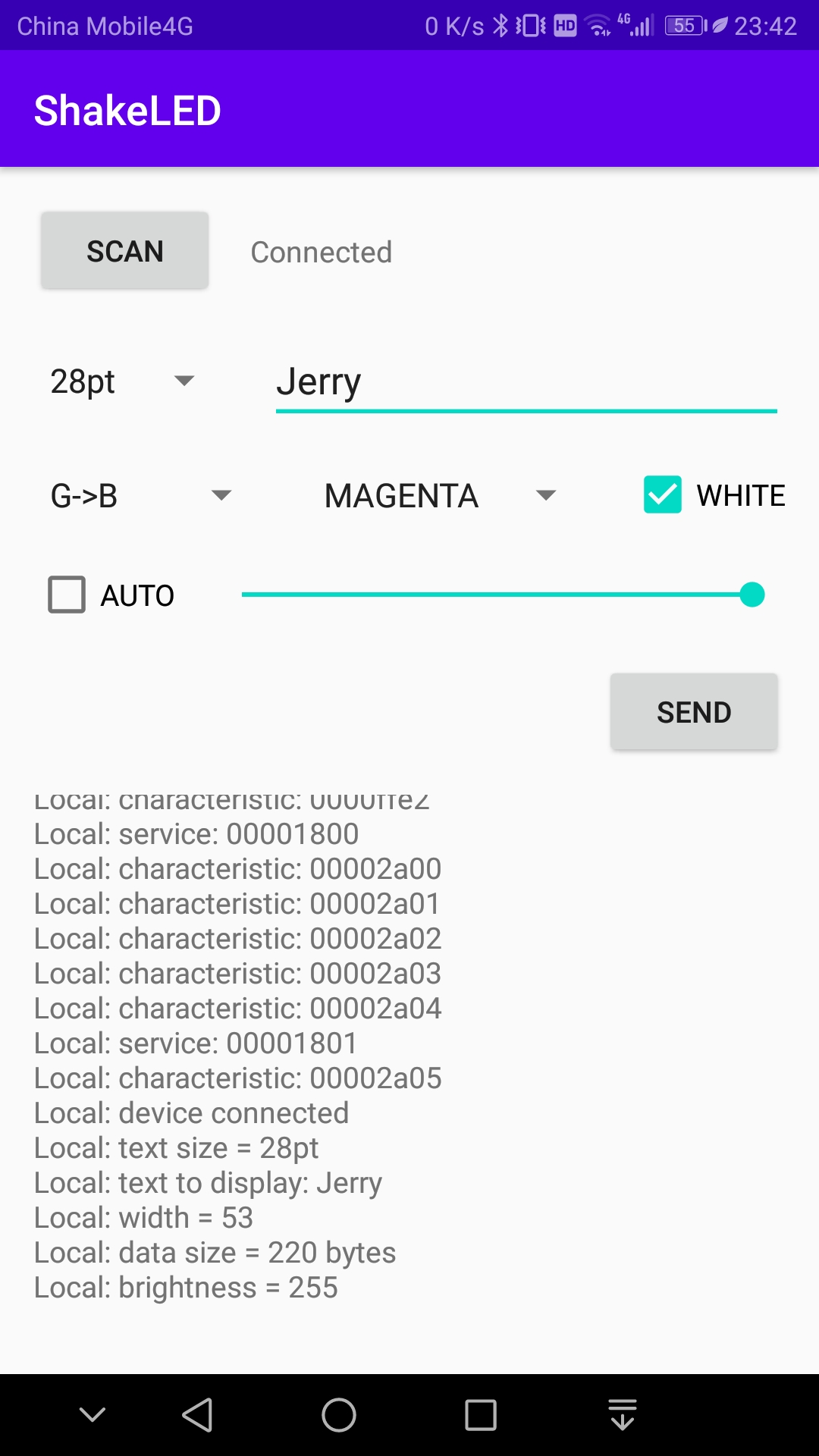### 后记

posted on 2020-06-25 22:24  jerry_fuyi  阅读(850)  评论(3编辑  收藏